#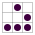computer/programs/useless/misc/pihome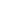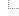computerprograms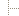usefuluselesssecunet_contest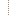entry_1entry_2entry_3entry_4entry_5entry_6japhjaph_1japh_2japh_3japh_4japh_5piethello_world_1hello_world_2Pietmiscpipolyglotgame_of_life``` \$|=3,141592;sub _ {print@_}sub o{_++\$O[0 ];_ 0for 1..\$#O}sub O{\$;=int \$=/10,'0/^ ^';if(\$;<9) {_\$_ for @O;;@O=() ;0}push @O,\$;;0 ,;push@ O,'.'if \$^==1; 0;if(\$; ==10){ print ,o,@O=( )}}\$?= 1000000 ;\$-=10* (q/@O= digits of pi =10/,1) *int(\$? /3)+1;\$ _=2for@ ,[0..\$-] ,;for\$^ (1..\$?){ \$"=\$-;\$O =0;until (\$"<0){\$/= 2*\$"+1;\$/= 10if\$/==1;\$==10*\$,[\$"]+\$O;\$, [\$"]=\$=%\$/;\$O=int(\$=/\$/ )*\$"--,10}O}o ``` Unable to load This Perl program computes the first million digits of pi (including the "3" before the decimal point) and prints them. You can modify the number of digits to be calculated by editing the "1000000" in the center of the code.The program implements the "Spigot Algorithm" which is described in this document together with several sample implementations far more efficient than mine. Be careful: the program is a number cruncher and will extremely slow down the performance of your computer. Moreover my implementation is awful slow (see below). Download the source. The output on the left was created on a computer with 2 processors Intel Dual Core Xeon 5150 2.66 GHz and 16 GB ram. It took 87 days, 14 hours, 12 minutes, 6 seconds to compute the one million digits on this machine! Note that the last digit should be "5" rather than "6", which results from a rounding error. Download the output.